Actions

(diff) ← Older revision | Latest revision (diff) | Newer revision → (diff)

A collection of four points(lying in a plane), no three of which lie on the same line, and the six lines connecting these points (cf. Fig.).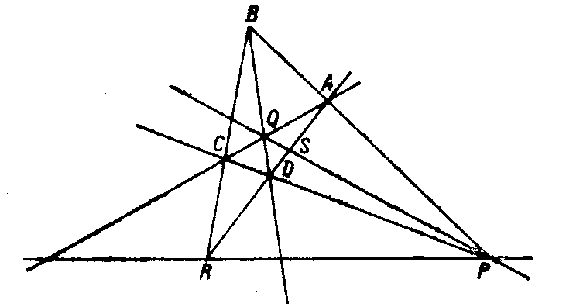Figure: q076010a

The points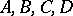are called the vertices, and the lines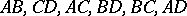are called the edges of the complete quadrangle. Edges that have no common vertex are called opposite; the points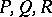of intersection of the opposite edges are called diagonal points.

Ifandare the points of intersection of the linewith the lines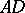and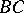, then the four points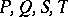form a harmonic quadruple of points. The dual figure to a quadrangle is called a quadrilateral — a collection of four lines (in a plane), no three of which contain a common point.